• 粒子群算法实例 matlab。 最近初学粒子群算法，自己按照自己的理解把每个步骤都解释了，然后每个步骤都解释的很清楚，很适合初学者观看，里面有matlab的代码，还有文档讲解，反正对于我一个初学者来说是能够看得懂得...
• 本文主要包含以下内容： 遗传算法和粒子群算法的程序设计的一般结构。主要介绍两类算法的程序设计中的主要思想。 介绍在Matlab编程中一些需要注意的细节。...一个遗传算法实例和一个粒子群算法实例
• 使用粒子群算法求最优解问题相较于传统算法计算速度非常快，全局搜索能力也很强；PSO对于种群大小不十分敏感，所以初始种群设为500-1000，速度影响也不大； 粒子群算法适用于连续函数极值问题，对于非线性、多峰问题...
• 这是粒子群算法的一个文件，含有相关讲解和代码，代码复制到matlab中就可以用了
• 粒子群算法（ Particle Swarm Optimization, PSO）最早是由Eberhart和Kennedy于1995年提出，它的基本概念源于对鸟群觅食行为的研究。鸟群中有个体和群体，个体和群体的信息是可以互通的。个体在随机搜寻食物的过程中...
粒子群算法（ Particle Swarm Optimization, PSO）最早是由Eberhart和Kennedy于1995年提出，它的基本概念源于对鸟群觅食行为的研究。鸟群中有个体和群体，个体和群体的信息是可以互通的。个体在随机搜寻食物的过程中，只要跟踪离食物最近的群体，就能最有效地找到食物。
1.一些基本概念
（1）粒子：优化问题的候选解，指鸟群中的一个个个体；
（2）位置：候选解所在的位置，即鸟群个体的位置；
（3）速度：粒子的移动速度；
（4）适应度：评价粒子优劣的值，一般为优化目标函数的数值；
（5）个体极值：单个粒子迄今为止找到的最佳位置，就鸟群觅食而言，是单个个体能够发现距离食物最近的个体；
（6）群体极值：所有粒子迄今为止找到的最佳位置。
2.大概流程及经典公式
根据这些概念，可以大概知道流程：1）初始粒子；2）计算适应度值；3）定义初始个体极值与群体极值；4）更新粒子位置与速度；5）更新个体极值和群体极值。
在实际问题的解决中，构建目标函数是最重要的，也是最难的。而粒子群算法中最经典的部分在于步骤4）（更新粒子位置与速度），其公式如下：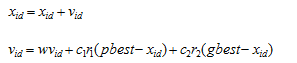xid——粒子的位置；
vid——粒子的移动速度；
w——惯性权重；
c1,c2——学习因子；
r1,r2——[0.1]中的随机数；
3.Matlab实现实例
（1）构建目标函数。这里使用只有两个参数的函数，这样便于画出三维图型。
function y=A11_01(x)

y=x(1)^2+x(2)^2-x(1)*x(2)-10*x(1)-4*x(2)+60;

首先从直观上看看这个函数：
x1=-15:1:15;
x2=-15:1:15;
[x1,x2]=meshgrid(x1,x2);
y=x1.^2+x2.^2-x1.*x2-10.*x1-4.*x2+60;
mesh(x1,x2,y);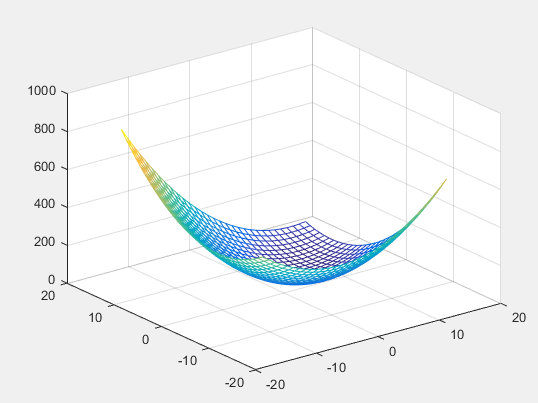图 1 目标函数的三维网格图
（2）整体实现代码
clear
clc

%绘制原图        图1目标函数的三维网格图
x1=-15:1:15;
x2=-15:1:15;
[x1,x2]=meshgrid(x1,x2);
y=x1.^2+x2.^2-x1.*x2-10.*x1-4.*x2+60;
mesh(x1,x2,y);
hold on;

%%预设参数
n=100; %粒子群的规模
d=2; %变量个数
c1=2;
c2=2;
w=0.9;%权重一般设为0.9
K=50; %迭代次数

%%分布粒子的取值范围及速度的取值范围
x=-10+20*rand(n,d);  %x在[-10,10]中取值
v=-5+10*rand(n,d); %v在[-5,5]中取值

%%计算适应度
fit=zeros(n,1);
for j=1:n
fit(j)=A11_01(x(j,:));
end
pbest=x;
ind=find(min(fit)==fit);
gbest=x(ind,:);
h=scatter3(x(:,1),x(:,2),fit,'o');  %图2 粒子的初始分布图

%%更新速度与位置
for i=1:K
for m=1:n
v(m,:)=w*v(m,:) + c1*rand*(pbest(m,:)-x(m,:)) + c2*rand*(gbest-x(m,:));%rand是[0,1]随机数

v(m,find(v(m,:)<-5))=-5;%这里发现速度小于-5时取-5
v(m,find(v(m,:)>5))=5;%这里发现速度大于5时取5

x(m,:)=x(m,:)+0.5*v(m,:);
x(m,find(x(m,:)<-10))=-10;%这里发现位置小于-10时取-10
x(m,find(x(m,:)>10))=10;%这里发现位置大于10时取10

%重新计算适应度
fit(m)=A11_01(x(m,:));
if x(m,:)<A11_01(pbest(m,:))
pbest(m,:)=x(m,:);
end
if A11_01(pbest(m,:))<A11_01(gbest)
gbest=pbest(m,:);
end
end
fitnessbest(i)=A11_01(gbest);
pause(0.01);    %为了直观，每更新一次，暂停0.01秒
h.XData=x(:,1);
h.YData=x(:,2);
h.ZData=fit;
end
% hold off;
% plot(fitnessbest);
% xlabel('迭代次数');

（3）粒子的初始分布图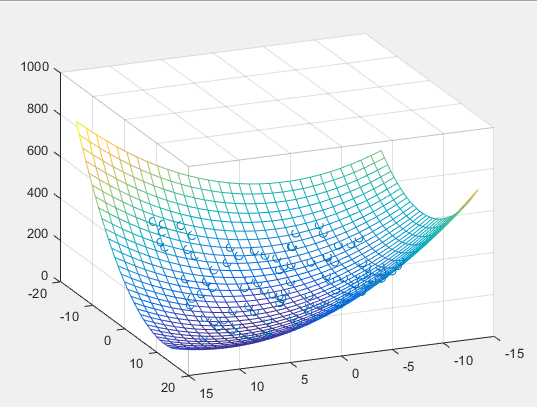图2 粒子的初始分布图
（4）粒子群移动图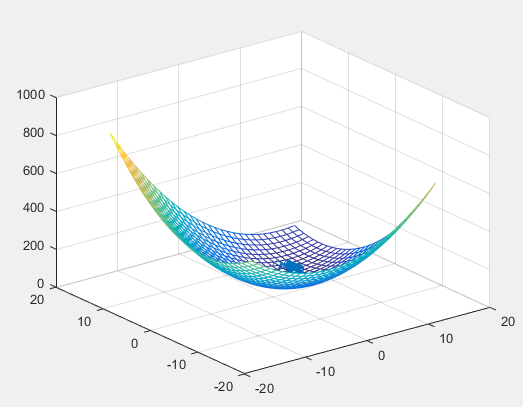图 3 粒子群移动图
（5）迭代过程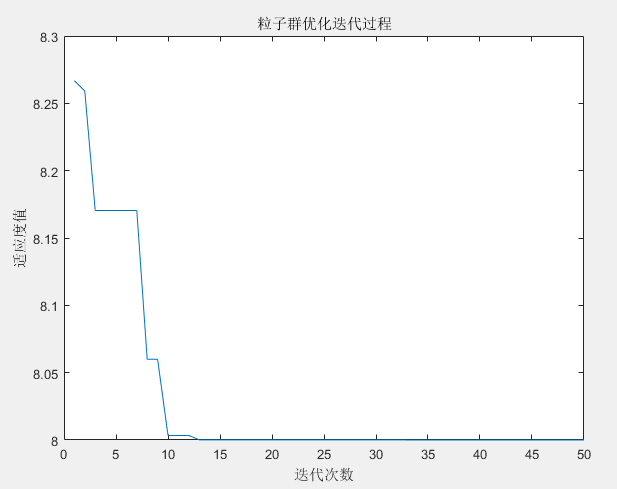图 4 迭代过程图
从图中可以看出，迭代次数基本上在13,14的时候就达到最优，即目标函数取得最小值为8.
4.总结
粒子群算法（PSO）在很多领域都有应用，本文提供的比较简单，函数来源于https://www.51zxw.net/show.aspx?id=71576&cid=641。
粒子群算法（PSO）在应用的过程中主要调整权重，学习因子，才能对解决的问题有所针对性。


展开全文• 粒子群算法作为MATLAB智能算法之一，其优点是简单好用
• 1.简介 物流分配中心选址问题是指...2.实例 现有20个客户点（序号为1-20）和7个可供配送选址点（序号为1-7），客户点有不同数量的货物需求，每个客户点的需求量如下表1所示。7个可供配送选址点的固定建设成本如表2所
1.简介
物流分配中心选址问题是指一定数量的客户,它们有不同数量的货物需求,有一定数量的备选中心作为配送中心。配送中心向客户提供货物的配送服务,现需要选择合适的配送中心位置（选址数量一定）或合适的配送中心数目和位置（选址数和位置同时优化）,目标是使得在客户的需求得到满足,并能在一定的约束下达到诸如路程最短、物流成本最小等目的。
2.实例
现有20个客户点（序号为1-20）和7个可供配送选址点（序号为1-7），客户点有不同数量的货物需求，每个客户点的需求量如下表1所示。7个可供配送选址点的固定建设成本如表2所示。现要求从7个可供配送选址点中选择4个点作为配送中心向客户提供货物的配送服务,请选择合适的选址位置和每个选址点对应的配送客户,目标是使得在客户的需求得到满足,并达到成本最小，请给出具体的配送选址方案。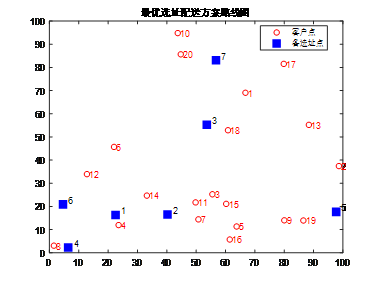目标：从7个备选址点中选出4个，并确定与20个客户点的对应关系，使总成本最小！
总成本分为两项：固定建设成本和运输成本，目标是使总成本最小
表1 20个客户点的需求量统计表
表2 7个可供配送选址点的固定建设成本统计表
3.编程及效果展示：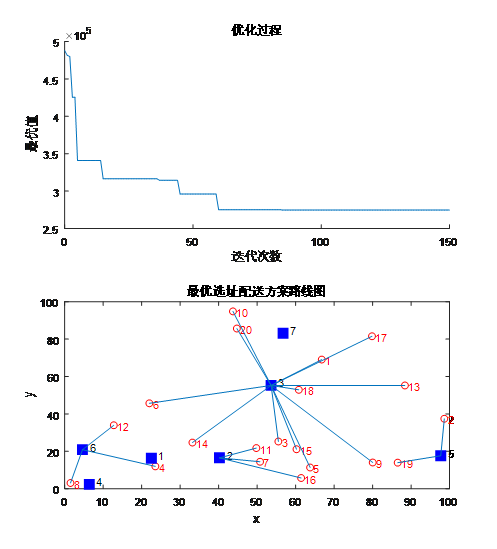视频展示优化过程：
MATLAB遗传算法求解物流配送中心选址问题优化实例

MATLAB粒子群算法求解物流配送中心选址问题优化实例

优化结果：
最优解:
优化结束！
选择的备选中心编号为：3  6  5  2
由备选中心3配送的需求点有：1   3   5   6   9  10  13  14  15  17  18  20
由备选中心6配送的需求点有：4   8  12
由备选中心5配送的需求点有：2  19
由备选中心2配送的需求点有：7  11  16微信公众号展开全文• end end % 便利整个种群，求整个种群最佳的适应度和位置 %% 粒子群迭代 % 更新速度并对速度进行边界处理 % 更新位置并对位置进行边界处理 % 计算新种群各个个体位置的适应度 % 新适应度与个体历史最佳适应度做比较 ...
%% 清空环境
clc;clear all;close all;
%% 设置种群参数
%   需要自行配置
sizepop = 500;                    % 初始种群个数
dim = 30;                          % 空间维数
ger =700;                       % 最大迭代次数
xlimit_max = 600*ones(dim,1);    % 设置位置参数限制(矩阵的形式可以多维)
xlimit_min = -600*ones(dim,1);
vlimit_max = 1*ones(dim,1);       % 设置速度限制
vlimit_min = -1*ones(dim,1);
c_1 = 0.8;                        % 惯性权重
c_2 = 0.5;                        % 自我学习因子
c_3 = 0.5;                        % 群体学习因子


%% 生成初始种群
%  首先随机生成初始种群位置
%  然后随机生成初始种群速度
%  然后初始化个体历史最佳位置，以及个体历史最佳适应度
%  然后初始化群体历史最佳位置，以及群体历史最佳适应度
for i=1:dim
for j=1:sizepop
pop_x(i,j) = xlimit_min(i)+(xlimit_max(i) - xlimit_min(i))*rand;
pop_v(i,j) = vlimit_min(i)+(vlimit_max(i) - vlimit_min(i))*rand;  % 初始种群的速度
end
end
gbest = pop_x;                                % 每个个体的历史最佳位置
fitness_gbest = fun(pop_x);                   % 每个个体的历史最佳适应度
zbest = pop_x(:,1);                           % 种群的历史最佳位置
fitness_zbest = fitness_gbest(1);             % 种群的历史最佳适应度

for j=1:sizepop
if fitness_gbest(j) < fitness_zbest       % 如果求最小值，则为<; 如果求最大值，则为>;
zbest = pop_x(:,j);
fitness_zbest=fitness_gbest(j);
end
end   % 便利整个种群，求整个种群最佳的适应度和位置


%% 粒子群迭代
%    更新速度并对速度进行边界处理
%    更新位置并对位置进行边界处理
%    计算新种群各个个体位置的适应度
%    新适应度与个体历史最佳适应度做比较
%    个体历史最佳适应度与种群历史最佳适应度做比较
%    再次循环或结束

iter = 1;                        %迭代次数
record = zeros(ger, 1);          % 记录器
while iter <= ger
for j=1:sizepop  % 对每个个体依次处理
%    更新速度并对速度进行边界处理
pop_v(:,j)= c_1 * pop_v(:,j) + c_2*rand*(gbest(:,j)-pop_x(:,j))+c_3*rand*(zbest-pop_x(:,j));% 速度更新

for i=1:dim
if  pop_v(i,j) > vlimit_max(i)
pop_v(i,j) = vlimit_max(i);
end
if  pop_v(i,j) < vlimit_min(i)
pop_v(i,j) = vlimit_min(i);
end
end

%    更新位置并对位置进行边界处理
pop_x(:,j) = pop_x(:,j) + pop_v(:,j);% 位置更新
for i=1:dim
if  pop_x(i,j) > xlimit_max(i)
pop_x(i,j) = xlimit_max(i);
end
if  pop_x(i,j) < xlimit_min(i)
pop_x(i,j) = xlimit_min(i);
end
end

%    计算新种群各个个体位置的适应度

fitness_pop(j) = fun(pop_x(:,j));                      % 当前个体的适应度

%    新适应度与个体历史最佳适应度做比较
if fitness_pop(j) < fitness_gbest(j)       % 如果求最小值，则为<; 如果求最大值，则为>;
gbest(:,j) = pop_x(:,j);               % 更新个体历史最佳位置
fitness_gbest(j) = fitness_pop(j);     % 更新个体历史最佳适应度
end

%    个体历史最佳适应度与种群历史最佳适应度做比较
if fitness_gbest(j) < fitness_zbest        % 如果求最小值，则为<; 如果求最大值，则为>;
zbest = gbest(:,j);                    % 更新群体历史最佳位置
fitness_zbest=fitness_gbest(j);        % 更新群体历史最佳适应度
end
end

record(iter) = fitness_zbest;%最优值记录

iter = iter+1;

end

%% 迭代结果输出
plot(record);title('收敛过程')
disp(['最优值：',num2str(fitness_zbest)]);
disp('变量取值：');
disp(zbest);


%% 目标函数
%    下列函数是一个多峰值的函数，在0向量处取得全局最小值0
function result = fun(X)
result = sum(X.^2)/4000 - prod(cos(X./((1:1:30).^0.5)')) + 1;
end




展开全文• 使用matlab编程，分为多个.m文件编写，包括支配关系选择，全局领导者选择，删除多于的非劣解，创建...套用算法只需要改动Mycost函数与主函数中粒子的取值与维度，即主函数中问题定义处与MOPSO设置处，其余不需要改动
• matlab 粒子群算法实例，改程序就一个简单的小例子，阐述了粒子群优化算法的构造过程
• 视频讲解+粒子群优化算法PPT+演讲稿+matlab算法实例
• 多个函数利用多种粒子群算法解决优化问题： 用二阶粒子群优化算法求解无约束优化问题 用二阶振荡粒子群优化算法求解无约束优化问题 用混沌粒子群优化算法求解无约束优化问题 用基于选择的粒子群优化算法求解无...
• 1、基本粒子群算法 假设在一个 D 维的目标搜索空间中，有 N 个粒子组成一个群落，其中第i 个 粒子表示为一个 D 维的向量： $$X_i=(x_{i1},x_{i2}, \cdots ,x_{iD}), \quad i=1,2, \cdots ,N \quad \text {(1)}$$...
目录

1、基本粒子群算法

2、基本粒子群算法流程

3、关键参数说明

3.1 粒子种群规模 $$N$$

3.2 惯性权重 $$w$$

3.3 加速常数 $$c_1$$ 和 $$c_2$$

3.4 最大速度 $$v_{max}$$

3.5 停止准则

3.6 邻域结构的设定

3.7 边界条件处理策略

4、MATLAB仿真实例

4.1 粒子群算法求解n元函数极值

粒子群算法求解n元函数极值问题MATLAB源程序

4.2 粒子群算法求解二元函数的极值

粒子群算法求解二元函数极值问题MATLAB源程序

5、粒子群算法的特点

6、其他种类的粒子群算法

6.1 标准粒子群算法

6.2 压缩因子粒子群算法

6.3 离散粒子群算法

1、基本粒子群算法

假设在一个 D 维的目标搜索空间中，有 N 个粒子组成一个群落，其中第 i 个 粒子表示为一个 D 维的向量：

$$X_i=(x_{i1},x_{i2}, \cdots ,x_{iD}), \quad i=1,2, \cdots ,N \quad \text {(1)}$$

第 i 个粒子的“飞行”速度也是一个 D 维的向量，记为:

$$V_i=(v_{i1},v_{i2}, \cdots ,v_{iD}), \quad i=1,2, \cdots ,N \quad \text {(2)}$$

第 i 个粒子迄今为止搜索到的最优位置称为个体极值，记为:

$$P_{best}=(p_{i1},p_{i2}, \cdots ,p_{iD}), \quad i=1,2, \cdots ,N \quad \text {(3)}$$

整个粒子群迄今为止搜索到的最优位置为全局极值，记为:

$$g_{best} = (g_1, g_2, \cdots ,g_D) \quad \text {(4)}$$

在找到这两个最优值时，粒子根据如下的式（5）和式（6）来更新自己的速度和位置：

$$v_{ij}(t+1) = v_{ij}(t) + c_1r_1(t)[p_{ij}(t) - x_{ij}(t)] + c_2r_2(t)[p_{gj}(t) - x_{ij}(t)] \quad \text {(5)}$$

$$x_{ij}(t+1) = x_{ij}(t) + v_{ij}(t+1) \quad \text {(6)}$$

其中：$$c_1$$和$$c_2$$为学习因子，也称加速常数；$$r_1$$和$$r_2$$为[0, 1]范围内的均匀随机数，增加了粒子飞行的随机性；$$v_{ij}$$是粒子的速度，$$v_{ij} \in [-v_{max}, v_{max}], v_{max}$$是常数，由用户设定来限制粒子的速度。

式（5）右边由三部分组成：第一部分为“惯性”或“动量”部分，反映了粒子的运动“习惯”，代表粒子有维持自己先前速度的趋势；第二部分为“认知”部分，反映了粒子对自身历史经验的记忆或回忆，代表粒子有向自身历史最佳位置逼近的趋势；第三部分为“社会”部分，反映了粒子间协同合作与知识共享的 群体历史经验，代表粒子有向群体或邻域历史最佳位置逼近的趋势。

2、基本粒子群算法流程

粒子群算法基于“种群”和“进化”的概念，通过个体间的协作与竞争，实现复杂空间最优解的搜索，其流程如下：

（1）初始化粒子群，包括群体规模 $$N$$，每个粒子的位置 $$x_i$$ 和速度 $$v_i$$。

（2）计算每个粒子的适应度值 $$fit[i]$$。

（3）对每个粒子，用它的适应度值 $$fit[i]$$ 和个体极值 $$p_{best}(i)$$ 比较。如果 $$fit[i] > p_{best}(i)$$，则用 $$fit[i]$$替换掉 $$p_{best}(i)$$。

（4）对每个粒子，用它的适应度值 $$fit[i]$$ 和全局极值 $$g_{best}$$ 比较。如果 $$fit[i] > g_{best}$$ 则用 $$fit[i]$$替换掉 $$g_{best}$$。

（5）迭代更新粒子的速度 $$v_i$$ 和位置 $$x_i$$。

（6）进行边界条件处理。

（7）判断算法终止条件是否满足：若是，则结束算法并输出优化结果；否则返回步骤（2）。

基本粒子群算法的算法流程图如下图所示：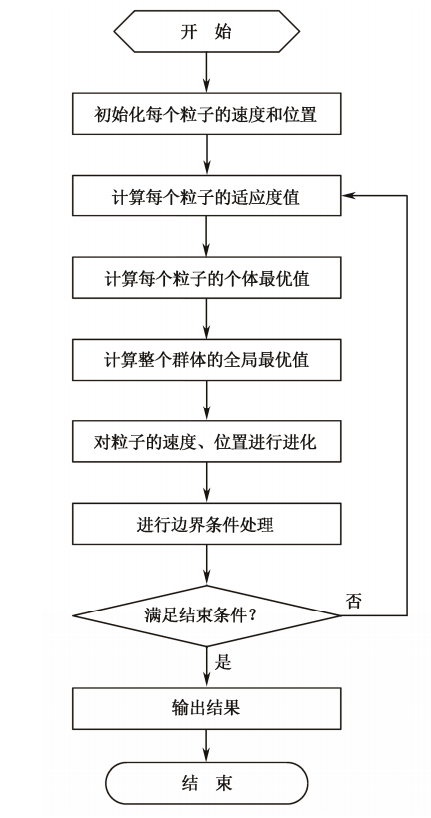3、关键参数说明

在粒子群优化算法中，控制参数的选择能够影响算法的性能和效率；如何选择合适的控制参数使算法性能最佳，是一个复杂的优化问题。在实际的优化问题中，通常根据使用者的经验来选取控制参数。粒子群算法的控制参数主要包括：粒子种群规模 $$N$$，惯性权重 $$w$$，加速常数 $$c_1$$ 和 $$c_2$$，最大速度 $$v_{max}$$，停止准则，邻域结构的设定，边界条件处理策略等。

3.1 粒子种群规模 $$N$$

粒子种群大小的选择视具体问题而定，但是一般设置粒子数为 20～50。对于大部分的问题 10 个粒子，已经可以取得很好的结果；不过对于比较难的问题或者特定类型的问题，粒子的数量可以取到 100 或 200。另外，粒子数目越大，算法搜索的空间范围就越大，也就更容易发现全局最优解；当然，算法运行的时间也越长。

3.2 惯性权重 $$w$$

惯性权重 $$w$$ 是标准粒子群算法中非常重要的控制参数，可以用来控制算法的开发和探索能力。惯性权重的大小表示了对粒子当前速度继承的多少。当惯性权重值较大时，全局寻优能力较强，局部寻优能力较弱；当惯性权重值较小时，全局寻优能力较弱，局部寻优能力较强。惯性权重的选择通常有固定权重和时变权重。固定权重就是选择常数作为惯性权重值，在进化过程中其值保持不变，一般取值为[0.8，1.2]；时变权重则是设定某一变化区间，在进化过程中按照某种方式逐步减小惯性权重。时变权重的选择包括变化范围和递减率。固定的惯性权重可以使粒子保持相同的探索和开发能力，而时变权重可以使粒子在进化的不同阶段拥有不同的探索和开发能力。

3.3 加速常数 $$c_1$$ 和 $$c_2$$

加速常数 $$c_1$$ 和 $$c_2$$ 分别调节向 $$p_{best}$$ 和 $$g_{best}$$ 方向飞行的最大步长，它们分别决定粒子个体经验和群体经验对粒子运行轨迹的影响，反映粒子群之间的信息交流。如果 $$c_1 = c_2 = 0$$，则粒子将以当前的飞行速度飞到边界。此时，粒子仅能搜索有限的区域，所以难以找到最优解。如果 $$c_1 = 0$$，则为“社会”模型，粒子缺乏认知能力，而只有群体经验，它的收敛速度较快，但容易陷入局部最优；如果 $$c_2 = 0$$，则为“认知”模型，没有社会的共享信息，个体之间没有信息的交互，所以找到最优解的概率较小。一个规模为 $$D$$ 的群体等价于运行了 $$N$$ 个各行其是的粒子，因此一般设置 $$c_1 = c_2$$。这样，个体经验和群体经验就有了同样重要的影响力，使得最后的最优解更精确。

3.4 最大速度 $$v_{max}$$

粒子的速度在空间中的每一维上都有一个最大速度限制值 $$v_{dmax}$$，用来对粒子的速度进行钳制，使速度控制在范围$$[-v_{dmax}，＋v_{dmax}]$$ 内，这决定问题空间搜索的力度，该值一般由用户自己设定。$$v_{max}$$ 是一个非常重要的参数，如果该值太大，则粒子们也许会飞过优秀区域；而如果该值太小，则粒子们可能无法对局部最优区域以外的区域进行充分的探测。它们可能会陷入局部最优，而无法移动足够远的距离而跳出局部最优，达到空间中更佳的位置。研究者指出，设定 $$v_{max}$$ 和调整惯性权重的作用是等效的，所以 $$v_{max}$$ 一般用于对种群的初始化进行设定，即将 $$v_{max}$$ 设定为每维变量的变化范围，而不再对最大速度进行细致的选择和调节。

3.5 停止准则

最大迭代次数、计算精度或最优解的最大停滞步数$$\Delta t$$（或可以接受的满意解）通常认为是停止准则，即算法的终止条件。根据具体的优化问题，停止准则的设定需同时兼顾算法的求解时间、优化质量和搜索效率等多方面性能。

3.6 邻域结构的设定

全局版本的粒子群算法将整个群体作为粒子的邻域，具有收敛速度快的优点，但有时算法会陷入局部最优。局部版本的粒子群算法将位置相近的个体作为粒子的邻域，收敛速度较慢，不易陷入局部最优值。实际应用中，可先采用全局粒子群算法寻找最优解的方向，即得到大致的结果，然后采用局部粒子群算法在最优点附近进行精细搜索。

3.7 边界条件处理策略

当某一维或若干维的位置或速度超过设定值时，采用边界条件处理策略可将粒子的位置限制在可行搜索空间内，这样能避免种群的膨胀与发散，也能避免粒子大范围地盲目搜索，从而提高了搜索效率。具体的方法有很多种，比如通过设置最大位置限制 $$x_{max}$$ 和最大速度限制 $$v_{max}$$，当超过最大位置或最大速度时，在范围内随机产生一个数值代替，或者将其设置为最大值，即边界吸收。

4、MATLAB仿真实例

4.1 粒子群算法求解n元函数极值

例1  计算函数 $$f(x)=\sum_{i=1}^n {{x_i}^2} \quad (-20 \leq x_i \leq 20)$$ 的最小值，其中个体 $$x$$ 的维数 $$n = 10$$。这是一个简单的平方和函数，只有一个极小点 $$x = (0，0，…，0)$$，理论最小值 $$f (0，0，…，0) = 0$$。

解：仿真过程如下：

（1）初始化群体粒子个数为 $$N = 100$$，粒子维数为 $$D = 10$$，最大迭代次数为 $$T = 200$$，学习因子 $$c_1 = c_2 = 1.5$$，惯性权重为 $$w = 0.8$$，位置最大值为 $$X_{max} = 20$$，位置最小值为 $$X_{min} = 20$$，速度最大值为 $$V_{max} = 10$$，速度最小值为 $$V_{min} = -10$$。

（2）初始化种群粒子位置 $$x$$ 和速度 $$v$$，粒子个体最优位置 $$p$$ 和最优值 $$p_{best}$$，以及粒子群全局最优位置 $$g$$ 和最优值 $$g_{best}$$。

（3）更新位置 $$x$$ 和速度值 $$v$$，并进行边界条件处理，判断是否替换粒子个体最优位置 $$p$$ 和最优值 $$p_{best}$$、粒子群全局最优位置 $$g$$ 和最优值 $$g_{best}$$。

（4）判断是否满足终止条件：若满足，则结束搜索过程，输出优化值；若不满足，则继续进行迭代优化。

优化结束后，其适应度进化曲线如下图所示，优化后的结果为 $$x = [0.6325 \quad 0.1572 \quad 0.4814 \quad 0.1091 \quad 0.3154 \quad 0.2236 \quad 0.3991 \quad 0.5907 \quad 0.0221 \quad 0.1172]×10^{-4}$$，函数 $$f(x)$$ 的最小值为 $$1.34×10^{-8}$$。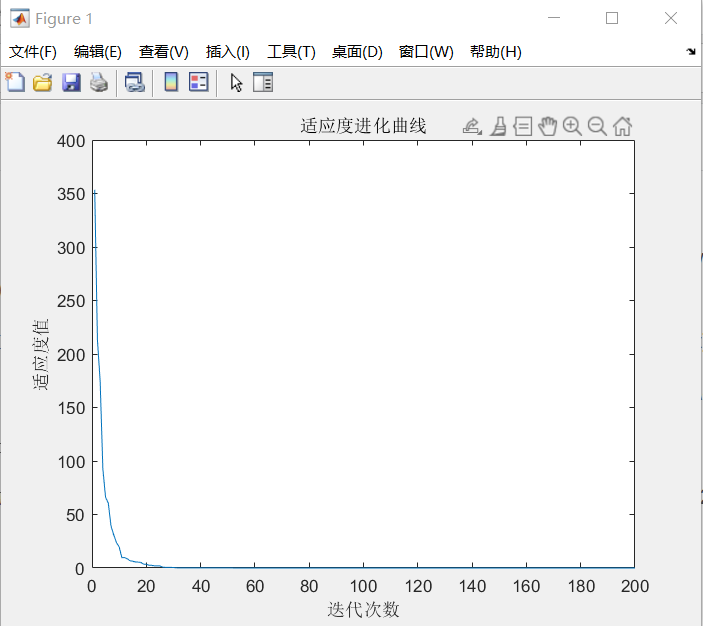粒子群算法求解n元函数极值问题MATLAB源程序

%%%%%%%%%%%%%%粒子群算法求函数极值%%%%%%%%%%%%%%%
%%%%%%%%%%%%%%%%%初始化%%%%%%%%%%%%%%%%%%%%%
clear all; %清除所有变量
close all; %清图
clc; %清屏
N = 100; %群体粒子个数
D = 10; %粒子维数
T = 200; %最大迭代次数
c1 = 1.5; %学习因子 1
c2 = 1.5; %学习因子 2
w = 0.8; %惯性权重
Xmax = 20; %位置最大值
Xmin = -20; %位置最小值
Vmax = 10; %速度最大值
Vmin = -10; %速度最小值
%%%%%%%%%%%初始化种群个体(限定位置和速度)%%%%%%%%%%%%%
x = rand(N,D) * (Xmax-Xmin)+Xmin;
v = rand(N,D) * (Vmax-Vmin)+Vmin;
%%%%%%%%%%%初始化个体最优位置和最优值%%%%%%%%%%%%%%%
p = x;
pbest = ones(N,1);
for i = 1:N
pbest(i) = func1(x(i,:));
end
%%%%%%%%%%%%%%初始化全局最优位置和最优值%%%%%%%%%%%%
g = ones(1,D);
gbest = inf;
for i = 1:N
if(pbest(i) < gbest)
g = p(i,:);
gbest = pbest(i);
end
end
gb = ones(1,T);
%%%%%%%%按照公式依次迭代直到满足精度或者迭代次数%%%%%%%%%
for i = 1:T
for j = 1:N
%%%%%%%%%%更新个体最优位置和最优值%%%%%%%%%%%%
if (func1(x(j,:)) < pbest(j))
p(j,:) = x(j,:);
pbest(j) = func1(x(j,:));
end
%%%%%%%%%%%更新全局最优位置和最优值%%%%%%%%%%%
if(pbest(j) < gbest)
g = p(j,:);
gbest = pbest(j);
end
%%%%%%%%%%%%%更新位置和速度值%%%%%%%%%%%%%
v(j,:) = w*v(j,:)+c1*rand*(p(j,:)-x(j,:))...
+c2*rand*(g-x(j,:));
x(j,:) = x(j,:)+v(j,:);
%%%%%%%%%%%%%%边界条件处理%%%%%%%%%%%%%%%
for ii = 1:D
if (v(j,ii) > Vmax) | (v(j,ii) < Vmin)
v(j,ii) = rand * (Vmax-Vmin)+Vmin;
end
if (x(j,ii) > Xmax) | (x(j,ii) < Xmin)
x(j,ii) = rand * (Xmax-Xmin)+Xmin;
end
end
end
%%%%%%%%%%%%%%记录历代全局最优值%%%%%%%%%%%%%
gb(i) = gbest;
end
g; %最优个体
gb(end); %最优值
figure
plot(gb)
xlabel('迭代次数');
ylabel('适应度值');
title('适应度进化曲线')

%%%%%%%%%%%%%%%%%%适应度函数%%%%%%%%%%%%%%%%
function result = func1(x)
summ = sum(x.^2);
result = summ;
end

4.2 粒子群算法求解二元函数的极值

例2  求函数 $$f(x，y) = 3cos(xy) + x + y^2$$ 的最小值，其中 $$x$$ 的取值范围为$$[-4，4]$$，$$y$$ 的取值范围为$$[-4，4]$$。这是一个有多个局部极值的函数，其函数值图形如下图所示。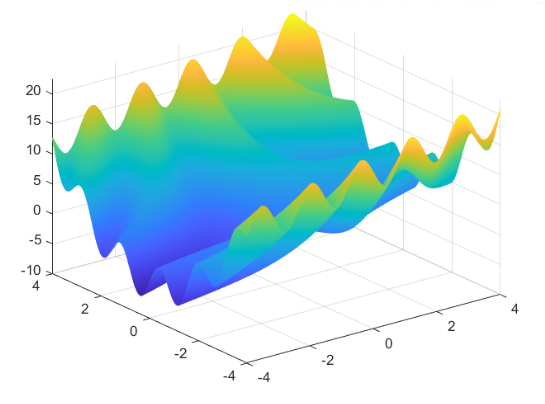解：仿真过程如下：

（1）初始化群体粒子个数为 $$N = 100$$，粒子维数为 $$D = 2$$，最大迭代次数为 $$T = 200$$，学习因子 $$c_1 = c_2 = 1.5$$，惯性权重最大值为 $$W_{max} = 0.8$$，惯性权重最小值为 $$W_{min} = 0.4$$，位置最大值为 $$X_{max} = 4$$，位置最小值为 $$X_{min} = -4$$，速度最大值为 $$V_{max} = 1$$，速度最大值为 $$V_{min} = -1$$。

（2）初始化种群粒子位置 $$x$$ 和速度 $$v$$，粒子个体最优位置 $$p$$ 和最优值 $$p_{best}$$，粒子群全局最优位置 $$g$$ 和最优值 $$g_{best}$$。

（3）计算动态惯性权重值 $$w$$，更新位置 $$x$$ 和速度值 $$v$$，并进行边界条件处理，判断是否替换粒子个体最优位置 $$p$$ 和最优值 $$p_{best}$$，以及粒子群全局最优位置 $$g$$ 和最优值 $$g_{best}$$。

（4）判断是否满足终止条件：若满足，则结束搜索过程，输出优化值；若不满足，则继续进行迭代优化。

优化结束后，其适应度进化曲线如下图所示。优化后的结果为：在 $$x=4.4395，y = 5$$ 时，函数 $$f(x)$$ 取得最小值$$-6.407$$。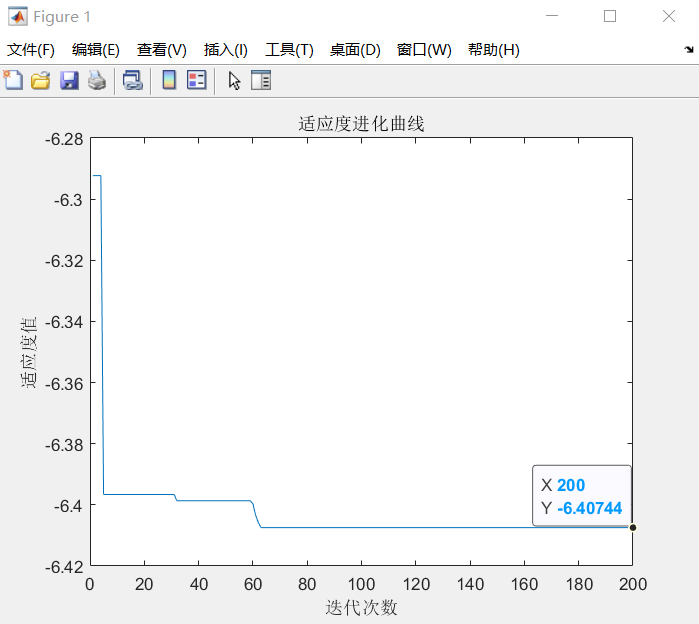粒子群算法求解二元函数极值问题MATLAB源程序

%%%%%%%%%%%%%%粒子群算法求函数极值%%%%%%%%%%%%%%%
%%%%%%%%%%%%%%%%%%初始化%%%%%%%%%%%%%%%%%%%
clear all; %清除所有变量
close all; %清图
clc; %清屏
N = 100; %群体粒子个数
D = 2; %粒子维数
T = 200; %最大迭代次数
c1 = 1.5; %学习因子 1
c2 = 1.5; %学习因子 2
Wmax = 0.8; %惯性权重最大值
Wmin = 0.4; %惯性权重最小值
Xmax = 4; %位置最大值
Xmin = -4; %位置最小值
Vmax = 1; %速度最大值
Vmin = -1; %速度最小值
%%%%%%%%%%%%初始化种群个体(限定位置和速度)%%%%%%%%%%%%
x = rand(N,D) * (Xmax-Xmin)+Xmin;
v = rand(N,D) * (Vmax-Vmin)+Vmin;
%%%%%%%%%%%%%初始化个体最优位置和最优值%%%%%%%%%%%%%
p = x;
pbest = ones(N,1);
for i = 1:N
pbest(i) = func2(x(i,:));
end
%%%%%%%%%%%%%初始化全局最优位置和最优值%%%%%%%%%%%%
g = ones(1,D);
gbest = inf;
for i = 1:N
if(pbest(i) < gbest)
g = p(i,:);
gbest = pbest(i);
end
end
gb = ones(1,T);
%%%%%%%%%按照公式依次迭代直到满足精度或者迭代次数%%%%%%%%
for i = 1:T
for j = 1:N
%%%%%%%%%更新个体最优位置和最优值%%%%%%%%%%%%%
if (func2(x(j,:)) < pbest(j))
p(j,:) = x(j,:);
pbest(j) = func2(x(j,:));
end
%%%%%%%%%%更新全局最优位置和最优值%%%%%%%%%%%%
if(pbest(j) < gbest)
g = p(j,:);
gbest = pbest(j);
end
%%%%%%%%%%%计算动态惯性权重值%%%%%%%%%%%%%%%
w = Wmax-(Wmax-Wmin)*i/T;
%%%%%%%%%%%%更新位置和速度值%%%%%%%%%%%%%%%
v(j,:) = w*v(j,:)+c1*rand*(p(j,:)-x(j,:))...
+c2*rand*(g-x(j,:));
x(j,:) = x(j,:)+v(j,:);
%%%%%%%%%%%%%%边界条件处理%%%%%%%%%%%%%%%
for ii = 1:D
if (v(j,ii) > Vmax) | (v(j,ii) < Vmin)
v(j,ii) = rand * (Vmax-Vmin)+Vmin;
end
if (x(j,ii) > Xmax) | (x(j,ii) < Xmin)
x(j,ii) = rand * (Xmax-Xmin)+Xmin;
end
end
end
%%%%%%%%%%%%%%记录历代全局最优值%%%%%%%%%%%%%%
gb(i) = gbest;
end
g; %最优个体
gb(end); %最优值
figure
plot(gb)
xlabel('迭代次数');
ylabel('适应度值');
title('适应度进化曲线')
%%%%%%%%%%%%%%%%%%适应度函数%%%%%%%%%%%%%%%%%
function value = func2(x)
value = 3*cos(x(1)*x(2))+x(1)+x(2)^2;
end

5、粒子群算法的特点

粒子群算法本质是一种随机搜索算法，它是一种新兴的智能优化技术。该算法能以较大概率收敛于全局最优解。实践证明，它适合在动态、多目标优化环境中寻优，与传统优化算法相比，具有较快的计算速度和更好的全局搜索能力。

（1）粒子群算法是基于群智能理论的优化算法，通过群体中粒子间的合作与竞争产生的群体智能指导优化搜索。与其他算法相比，粒子群算法是一种高效的并行搜索算法。

（2）粒子群算法与遗传算法都是随机初始化种群，使用适应值来评价个体的优劣程度和进行一定的随机搜索。但粒子群算法根据自己的速度来决定搜索，没有遗传算法的交叉与变异。与进化算法相比，粒子群算法保留了基于种群的全局搜索策略，但是其采用的速度位移模型操作简单，避免了复杂的遗传操作。

（3）由于每个粒子在算法结束时仍保持其个体极值，即粒子群算法除了可以找到问题的最优解外，还会得到若干较好的次优解，因此将粒子群算法用于调度和决策问题可以给出多种有意义的方案。

（4）粒子群算法特有的记忆使其可以动态地跟踪当前搜索情况并调整其搜索策略。另外，粒子群算法对种群的大小不敏感，即使种群数目下降时，性能下降也不是很大。

6、其他种类的粒子群算法

6.1 标准粒子群算法

引入研究粒子群算法经常用到的两个概念：一是“探索”，指粒子在一定程度上离开原先的搜索轨迹，向新的方向进行搜索，体现了一种向未知区域开拓的能力，类似于全局搜索；二是“开发”，指粒子在一定程度上继续在原先的搜索轨迹上进行更细一步的搜索，主要指对探索过程中所搜索到的区域进行更进一步的搜索。探索是偏离原来的寻优轨迹去寻找一个更好的解，探索能力是一个算法的全局搜索能力。开发是利用一个好的解，继续原来的寻优轨迹去搜索更好的解，它是算法的局部搜索能力。如何确定局部搜索能力和全局搜索能力的比例，对一个问题的求解过程很重要。1998 年，Y. H. Shi 提出了带有惯性权重的改进粒子群算法，由于该算法能够保证较好的收敛效果，所以被默认为标准粒子群算法。其进化过程为：

$$v_{ij}(t+1) = w \cdot v_{ij}(t) + c_1r_1(t)[p_{ij}(t) - x_{ij}(t)] + c_2r_2(t)[p_{gj}(t) - x_{ij}(t)] \quad \text {(7)}$$

$$x_{ij}(t+1) = x_{ij}(t) + v_{ij}(t+1) \quad \text {(8)}$$

在式（7）中，第一部分表示粒子先前的速度，用于保证算法的全局收敛性能；第二部分、第三部分则使算法具有局部收敛能力。可以看出，式（7）中惯性权重 w 表示在多大程度上保留原来的速度：w 较大，则全局收敛能力较强，局部收敛能力较弱；w 较小，则局部收敛能力较强，全局收敛能力较弱。

当 w = 1 时，式（7）与式（5）完全一样，表明带惯性权重的粒子群算法是基本粒子群算法的扩展。实验结果表明：w 在 0.8～1.2 之间时，粒子群算法有更快的收敛速度；而当 w > 1.2 时，算法则容易陷入局部极值。

另外，在搜索过程中可以对 w 进行动态调整：在算法开始时，可给 w 赋予较大正值，随着搜索的进行，可以线性地使 w 逐渐减小，这样可以保证在算法开始时，各粒子能够以较大的速度步长在全局范围内探测到较好的区域；而在搜索后期，较小的 w 值则保证粒子能够在极值点周围做精细的搜索，从而使算法有较大的概率向全局最优解位置收敛。对 w 进行调整，可以权衡全局搜索和局部搜索能力。目前，采用较多的动态惯性权重值是 Shi 提出的线性递减权值策略，其表达式如下：

$$w = w_{max} - \frac {(w_{max} - w_{min}) \cdot t} {T_{max}} \quad \text {(9)}$$

其中，$$T_{max}$$ 表示最大进化代数；$$w_{min}$$ 表示最小惯性权重；$$w_{max}$$ 表示最大惯性权重；$$t$$ 表示当前迭代次数。在大多数的应用中 $$w_{max} = 0.9，w_{min} = 0.4$$。

6.2 压缩因子粒子群算法

Clerc 提出利用约束因子来控制系统行为的最终收敛，该方法可以有效搜索不同的区域，并且能得到高质量的解。压缩因子法的速度更新公式为：

$$v_{ij}(t+1) = \lambda \cdot v_{ij}(t) + c_1r_1(t)[p_{ij}(t) - x_{ij}(t)] + c_2r_2(t)[p_{gj}(t) - x_{ij}(t)] \quad \text {(10)}$$

$$\lambda = \frac {2} {\mid 2 - \varphi - \sqrt{(\varphi ^2 - 4 \varphi )} \mid} \quad \text {(11)}$$

$$\varphi = c_1 + c_2 \quad \text {(12)}$$

其中，$$\lambda$$ 为压缩因子。实验结果表明，与使用惯性权重的粒子群优化算法相比，使用具有约束因子的粒子群算法具有更快的收敛速度。

6.3 离散粒子群算法

基本的粒子群算法是在连续域中搜索函数极值的有力工具。继基本粒子群算法之后，Kennedy 和 Eberhart 又提出了一种离散二进制版的粒子群算法。在此离散粒子群方法中，将离散问题空间映射到连续粒子运动空间，并适当修改粒子群算法来求解，在计算上仍保留经典粒子群算法速度-位置更新运算规则。粒子在状态空间的取值和变化只限于 0 和 1 两个值，而速度的每一维 $$v_{ij}$$ 代表位置每一位 $$x_{ij}$$ 取值为 1 的可能性。因此，在连续粒子群中的 $$v_{ij}$$ 更新公式依然保持不变，但是 $$p_{best}$$ 和 $$g_{best}$$ 只在 $$[0，1]$$ 内取值。其位置更新等式表示如下：

$$s(v_{ij}) = \frac {1} {1 + e^{-v_{ij}}} \quad \text {(13)}$$

$$x_{ij} = \begin{cases} 1, & \text {r < s(v_{ij})} \\ 0, & \text{其他} \end{cases} \quad \text {(14)}$$

式中，$$r$$ 是从 $$U(0，1)$$分布中产生的随机数。

往期相关博客：

1、蚁群算法小结及算法实例（附Matlab代码）

2、遗传算法小结及算法实例（附Matlab代码）

3、免疫算法小结及算法实例（附Matlab代码）

展开全文算法
• 最近在做毕业设计，要用量子粒子群算法实现优化，自己看的一头雾水，哪位好心人有相关的案例可以分享一下吗？拜托了！
• 实现粒子群算法matlab代码，包含3个文件，主要是用一个实例加以说明
• 遗传算法简单实例matlab ...粒子群算法简单实例matlab https://github.com/Zoyeeee/Particle-swarm-optimization-PSO- 蚁群算法简单实例matlab https://github.com/Zoyeeee/ASO-
• 最后,采用matlab语言编程实现了基于改进的粒子群优化算法的测试用例自动生成算法,用具体实例对其进行了实验,并对结果数据进行了分析。 本文提出的基于改进的粒子群优化算法的测试用例自动生成算法具有简单、易实现...
• 基于Ackley's function的，差分进化算法和粒子群算法的比较。 以下是matlab源码。 %%%%%%%%%%%% %%%%%%%%%%%% Ackley's function clear all; %清除所有变量 close all; %清图 clc; %清屏 x=-5:0.01:5; y=-5:...
• 标准粒子群算法的进化过程如下 matlab源码 %该脚本要命名为func2.m %%%%%%%%%%%%%%%%%%%%%适应度函数%%%%%%%%%%%%%%%%%%%%%%% function value=func2(x) value=sin(x(1)*x(1)+x(2))+cos(5*x(1))+x(1)+x(2); %...
• MATLAB编写PSO算法及实例 1.1 粒子群算法 PSO从这种模型中得到启示并用于解决优化问题PSO 中每个优化问题的潜在解都是搜索空间中的一只鸟称之为粒子所有的粒子都有一个由被优化的函数决定的适值( fitness value) ...
• 1995年，美国社会心理学家James Kennedy和电气工程师Russell Eberhart共同提出了粒子群算法（Particle Swarm Optimization，PSO），该算法的提出是受对鸟类群体行为进行建模与仿真的研究结果的启发。 粒子群优化...
• 文章目录问题描述仿真过程matlab源码 问题描述 仿真过程 matlab源码 %该脚本要命名为func1.m %%%%%%%%%%%%%%%%%%%适应度函数%%%%%%%%%%%%%%...%%%%%%%%%%%%%%%%%粒子群算法求函数极值%%%%%%%%%%%%%%%%%%%%%%%%%% %%%%# matlab粒子群算法实例matlab 订阅